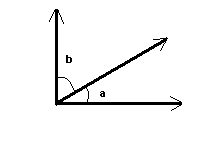Angles in Geometry

# Angles in Geometry

Definitions and properties of angles in geometry.

## An Angle in Geometry

An angle is the rotation required to superimpose one of two intersecting lines on the other.## Right Angle

A right angle is an angle with measure equal to 90 degrees.## Straight Angle

A straight angle is an angle with measure equal to 180 degrees.## An Acute Angle

An acute angle is an angle with a measure between 0 and 90 degrees.## An Obtuse Angle

An obtuse angle is an angle with a measure between 90 and 180 degrees.## Complementary Angles

Two angles are complementary if the sum of their measures is equal to 90 degree.Example: angles a and b with measures a = 19� and b = 71� are complementary since a + b = 90�

## Supplementary Angles

Two angles are supplementary if the sum of their measures is equal to 180 degrees.Example: angles a and b with measures a = 122.1� and b = 57.9� are supplementary since a + b = 180�

## Exercises (see solutions below)

Given angles a, b, c, d, e and f with measures
a = 21�
b = 90.1�
c = 90�
d = 134.2�
e = 69�
f = 45.8�
1 ) Which of these angles are acute?
2 ) Which of these angles are obtuse?
3 ) Which pairs of angles are complementary?
4 ) Which pairs of angles are supplementary?

1 ) a, e and f
2 ) b and d.
3 ) a - e
4 ) d - f

## More References and Links to Geometry Tutorials

Questions on Angles with Solutions and Explanations
Geometry Tutorials and Problems